Python courses Archives - DexLab Analytics | Big Data Hadoop SAS R Analytics Predictive Modeling & Excel VBA

## MongoDB Basics Part-II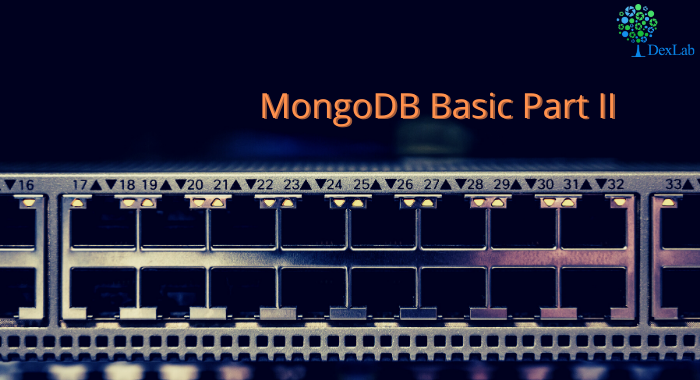In our previous blog we discussed about few of the basic functions of MQL like .find() , .count() , .pretty() etc. and in this blog we will continue to do the same. At the end of the blog there is a quiz for you to solve, feel free to test your knowledge and wisdom you have gained so far.

Given below is the list of functions that can be used for data wrangling:-

1. updateOne() :- This function is used to change the current value of a field in a single document.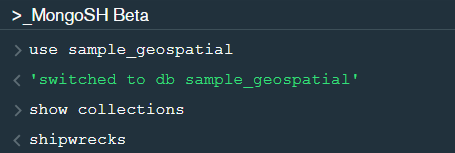After changing the database to “sample_geospatial” we want to see what the document looks like? So for that we will use .findOne() function.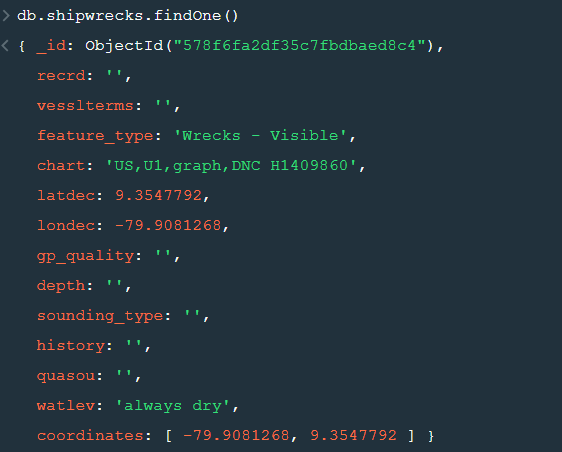Now lets update the field value of “recrd” from ‘ ’ to “abc” where the “feature_type” is ‘Wrecks-Visible’.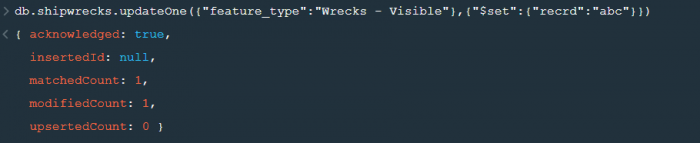Now within the .updateOne() funtion any thing in the first part of { } is the condition on the basis of which we want to update the given document and the second part is the changes which we want to make. Here we are saying that set the value as “abc” in the “recrd” field . In case you wanted to increase the value by a certain number ( assuming that the value is integer or float) you can use “\$inc” instead.

2. updateMany() :- This function updates many documents at once based on the condition provided.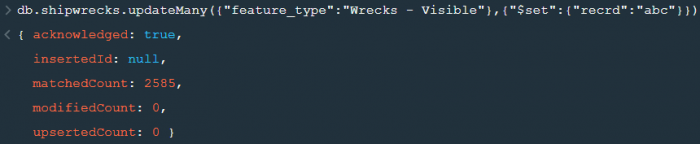3. deleteOne() & deleteMany() :- These functions are used to delete one or many documents based on the given condition or field.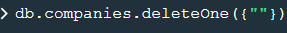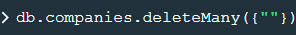4. Logical Operators :-

“\$and” : It is used to match all the conditions.

“\$or” : It is used to match any of the conditions.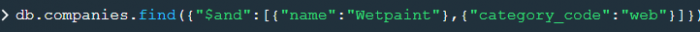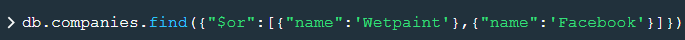The first code matches both the conditions i.e. name should be “Wetpaint” and “category_code” should be “web”, whereas the second code matches any one of the conditions i.e. either name should be “Wetpaint” or “Facebook”. Try these codes and see the difference by yourself.

So, with that we come to the end of the discussion on the MongoDB Basics. Hopefully it helped you understand the topic, for more information you can also watch the video tutorial attached down this blog. The blog is designed and prepared by Niharika Rai, Analytics Consultant, DexLab Analytics DexLab Analytics offers machine learning courses in Gurgaon. To keep on learning more, follow DexLab Analytics blog.

.

## Introduction to MongoDB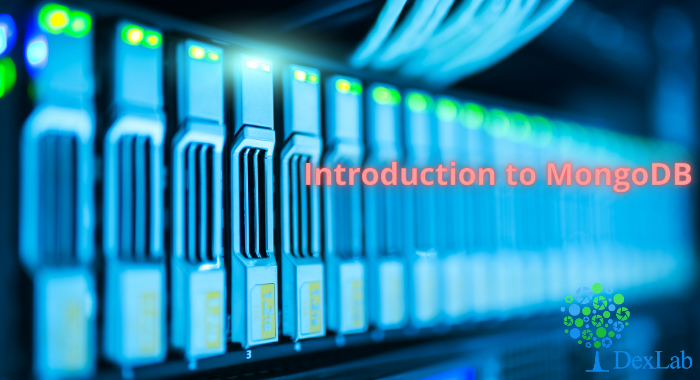MongoDB is a document based database program which was developed by MongoDB Inc. and is licensed under server side public license (SSPL). It can be used across platforms and is a non-relational database also known as NoSQL, where NoSQL means that the data is not stored in the conventional tabular format and is used for unstructured data as compared to SQL and that is the major difference between NoSQL and SQL.
MongoDB stores document in JSON or BSON format. JSON also known as JavaScript Object notation is a format where data is stored in a key value pair or array format which is readable for a normal human being whereas BSON is nothing but the JSON file encoded in the binary format which is quite hard for a human being to understand.
Structure of MongoDB which uses a query language MQL(Mongodb query language):-
Databases:- Databases is a group of collections.
Collections:- Collection is a group fields.
Fields:- Fields are nothing but key value pairs
Just for an example look at the image given below:-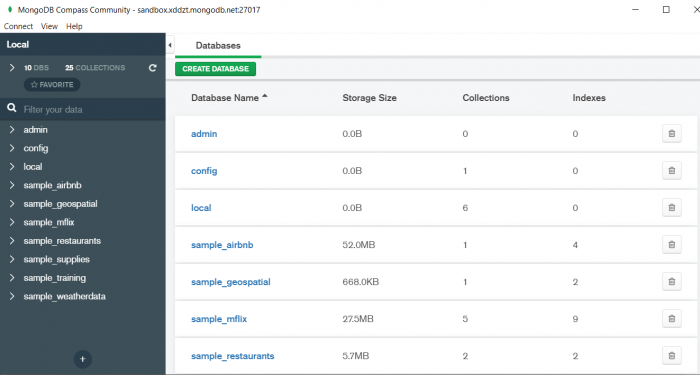Here I am using MongoDB Compass a tool to connect to Atlas which is a cloud based platform which can help us write our queries and start performing all sort of data extraction and deployment techniques. You can download MongoDB Compass via the given link https://www.mongodb.com/try/download/compassIn the above image in the red box we have our databases and if we click on the “sample_training” database we will see a list of collections similar to the tables in sql.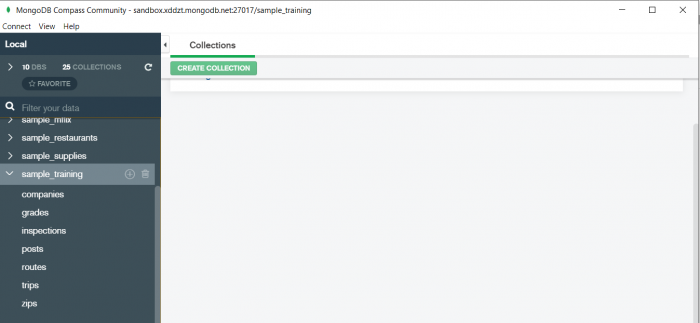Now lets write our first query and see what data in “companies” collection looks like but before that select the “companies” collection.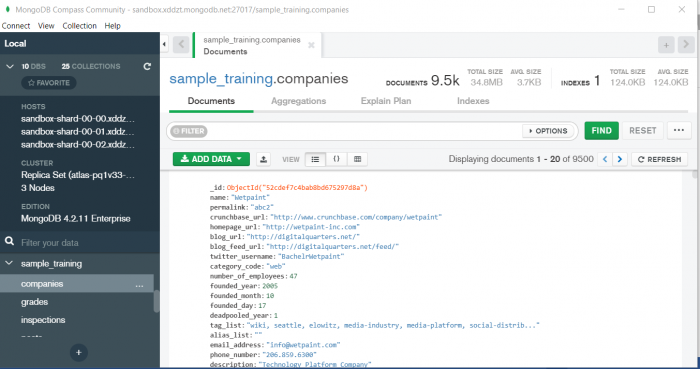Now in our filter cell we can write the following query:-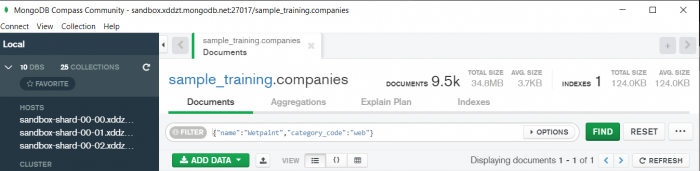In the above query “name” and “category_code” are the key values also known as fields and “Wetpaint” and “web” are the pair values on the basis of which we want to filter the data.
What is cluster and how to create it on Atlas?
MongoDB cluster also know as sharded cluster is created where each collection is divided into shards (small portions of the original data) which is a replica set of the original collection. In case you want to use Atlas there is an unpaid version available with approximately 512 mb space which is free to use. There is a pre-existing cluster in MongoDB named Sandbox , which currently I am using and you can use it too by following the given steps:-
2. Click on “Create an Organization”.
3. Write the organization name “MDBU”.
4. Click on “Create Organization”.
5. Click on “New Project”.
6. Name your project M001 and click “Next”.
7. Click on “Build a Cluster”.
8. Click on “Create a Cluster” an option under which free is written.
9. Click on the region closest to you and at the bottom change the name of the cluster to “Sandbox”.
10. Now click on connect and click on “Allow access from anywhere”.
11. Create a Database User and then click on “Create Database User”.
12. Click on “Close” and now load your sample as given below :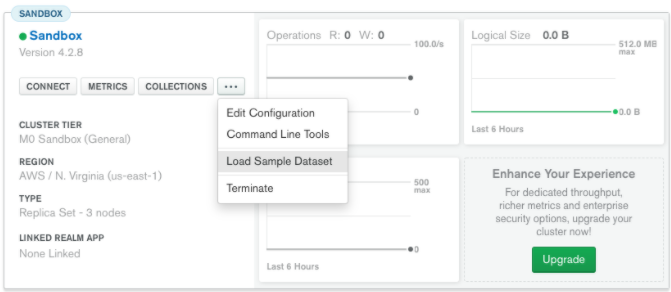13. Click on collections once the sample is loaded and now you can start using the filter option in a similar way as in MongoDB Compass
In my next blog I’ll be sharing with you how to connect Atlas with MongoDB Compass and we will also learn few ways in which we can write query using MQL.

So, with that we come to the end of the discussion on the MongoDB. Hopefully it helped you understand the topic, for more information you can also watch the video tutorial attached down this blog. The blog is designed and prepared by Niharika Rai, Analytics Consultant, DexLab Analytics DexLab Analytics offers machine learning courses in Gurgaon. To keep on learning more, follow DexLab Analytics blog.

.

## ARMA- Time Series Analysis Part 4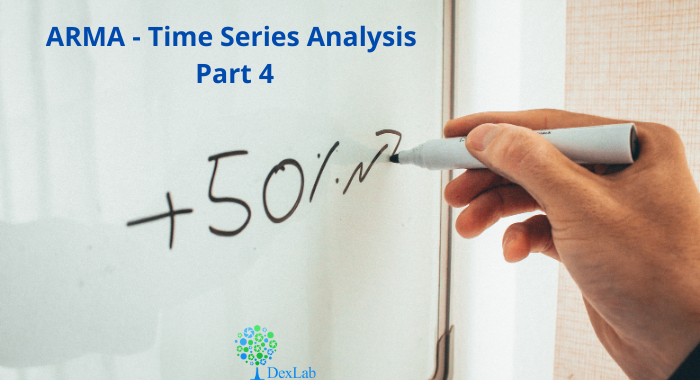ARMA(p,q) model in time series forecasting is a combination of Autoregressive  Process also known as AR Process and Moving Average (MA) Process where p corresponds to the autoregressive part and q corresponds to the moving average part.Autoregressive Process (AR) :- When the value of Yt in a time series data is regressed over its own past value then it is called an autoregressive process where p is the order of lag into consideration.Where,

Yt = observation which we need to find out.

α1= parameter of an autoregressive model

Yt-1= observation in the previous period

ut= error term

The equation above follows the first order of autoregressive process or AR(1) and the value of p is 1. Hence the value of Yt in the period ‘t’ depends upon its previous year value and a random term.

Moving Average (MA) Process :- When the value of Yt of order q in a time series data depends on the weighted sum of current and the q recent errors i.e. a linear combination of error terms then it is called a moving average process which can be written as :-

yt = observation which we need to find out

α= constant term

βut-q= error over the period q .

ARMA (Autoregressive Moving Average) Process :-The above equation shows that value of Y in time period ‘t’ can be derived by taking into consideration the order of lag p which in the above case is 1 i.e. previous year’s observation and the weighted average of the error term over a period of time q which in case of the above equation is 1.

How to decide the value of p and q?

Two of the most important methods to obtain the best possible values of p and q are ACF and PACF plots.

ACF (Auto-correlation function) :- This function calculates the auto-correlation of the complete data on the basis of lagged values which when plotted helps us choose the value of q that is to be considered to find the value of Yt. In simple words how many years residual can help us predict the value of Yt can obtained with the help of ACF, if the value of correlation is above a certain point then that amount of lagged values can be used to predict Yt.

Using the stock price of tesla between the years 2012 and 2017 we can use the .acf() method in python to obtain the value of p.

.DataReader() method is used to extract the data from web.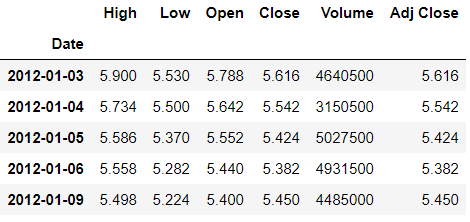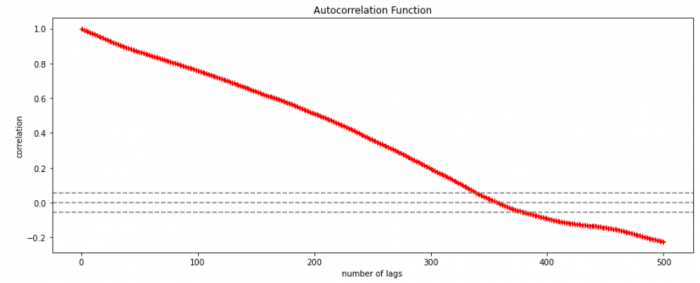The above graph shows that beyond the lag 350 the correlation moved towards 0 and then negative.

PACF (Partial auto-correlation function) :- Pacf helps find the direct effect of the past lag by removing the residual effect of the lags in between. Pacf helps in obtaining the value of AR where as acf helps in obtaining the value of MA i.e. q. Both the methods together can be use find the optimum value of p and q in a time series data set.

Lets check out how to apply pacf in python.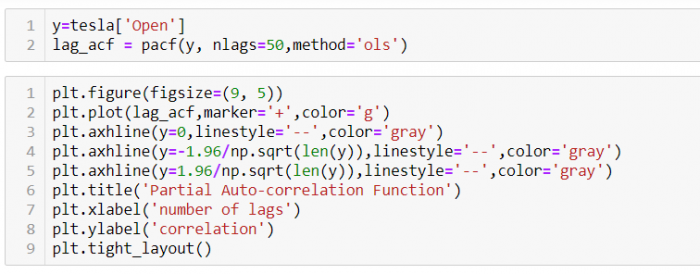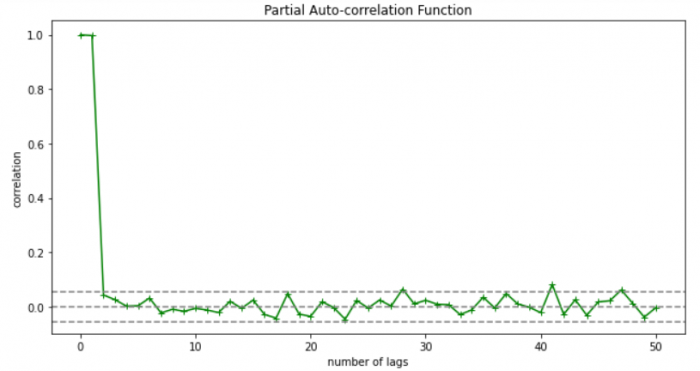As you can see in the above graph after the second lag the line moved within the confidence band therefore the value of p will be 2.

So, with that we come to the end of the discussion on the ARMA Model. Hopefully it helped you understand the topic, for more information you can also watch the video tutorial attached down this blog. The blog is designed and prepared by Niharika Rai, Analytics Consultant, DexLab Analytics DexLab Analytics offers machine learning courses in Gurgaon. To keep on learning more, follow DexLab Analytics blog.

.

## Autocorrelation- Time Series – Part 3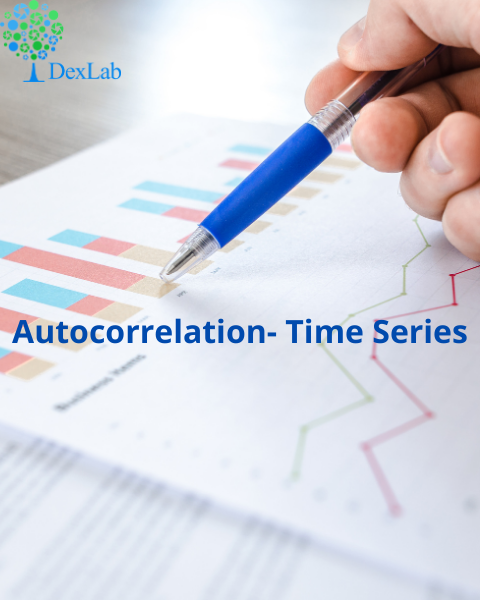Autocorrelation is a special case of correlation. It refers to the relationship between successive values of the same variables .For example if an individual with a consumption pattern:-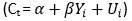spends too much in period 1 then he will try to compensate that in period 2 by spending less than usual. This would mean that Ut is correlated with Ut+1 . If it is plotted the graph will appear as follows :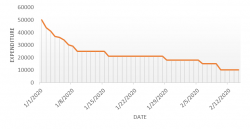Positive Autocorrelation : When the previous year’s error effects the current year’s error in such a way that when a graph is plotted the line moves in the upward direction or when the error of the time t-1 carries over into a positive error in the following period it is called a positive autocorrelation.
Negative Autocorrelation : When the previous year’s error effects the current year’s error in such a way that when a graph is plotted the line moves in the downward direction or when the error of the time t-1 carries over into a negative error in the following period it is called a negative autocorrelation.

Now there are two ways of detecting the presence of autocorrelation
By plotting a scatter plot of the estimated residual (ei) against one another i.e. present value of residuals are plotted against its own past value.If most of the points fall in the 1st and the 3rd quadrants , autocorrelation will be positive since the products are positive.If most of the points fall in the 2nd and 4th quadrant , the autocorrelation will be negative, because the products are negative.
By plotting ei against time : The successive values of ei are plotted against time would indicate the possible presence of autocorrelation .If e’s in successive time show a regular time pattern, then there is autocorrelation in the function. The autocorrelation is said to be negative if successive values of ei changes sign frequently.
First Order of Autocorrelation (AR-1)
When t-1 time period’s error affects the error of time period t (current time period), then it is called first order of autocorrelation.
AR-1 coefficient p takes values between +1 and -1
The size of this coefficient p determines the strength of autocorrelation.
A positive value of p indicates a positive autocorrelation.
A negative value of p indicates a negative autocorrelation
In case if p = 0, then this indicates there is no autocorrelation.
To explain the error term in any particular period t, we use the following formula:-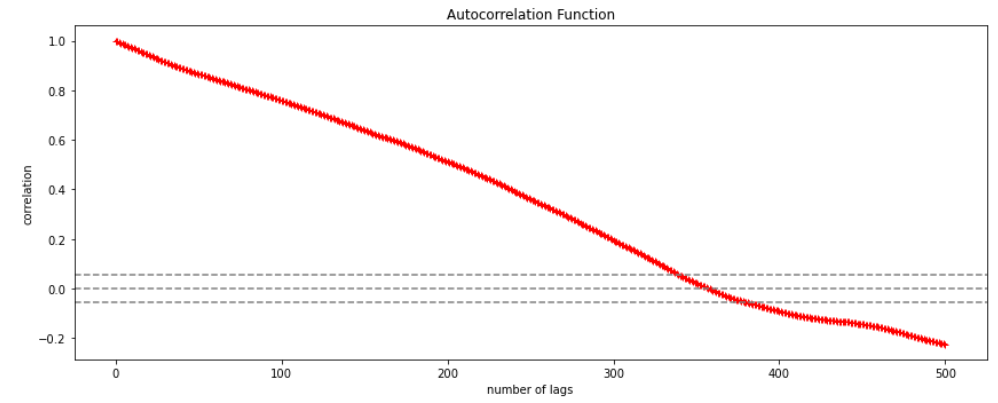Where Vt= a random term which fulfills all the usual assumptions of OLS
How to find the value of p?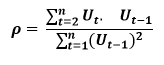One can estimate the value of ρ by applying the following formula :-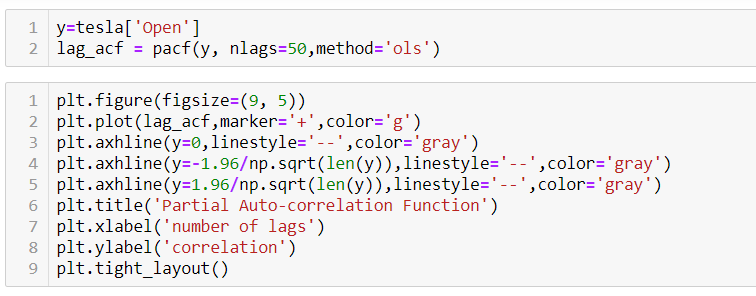## Time Series Analysis & Modelling with Python (Part II) – Data Smoothing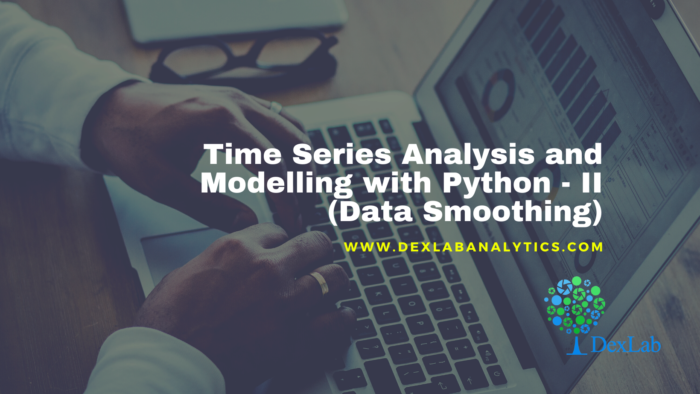Data Smoothing is done to better understand the hidden patterns in the data. In the non- stationary processes, it is very hard to forecast the data as the variance over a period of time changes, therefore data smoothing techniques are used to smooth out the irregular roughness to see a clearer signal.

In this segment we will be discussing two of the most important data smoothing techniques :-

• Moving average smoothing
• Exponential smoothing

Moving average smoothing

Moving average is a technique where subsets of original data are created and then average of each subset is taken to smooth out the data and find the value in between each subset which better helps to see the trend over a period of time.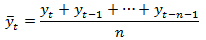Lets take an example to better understand the problem.

Suppose that we have a data of price observed over a period of time and it is a non-stationary data so that the tend is hard to recognize.

 QTR (quarter) Price 1 10 2 11 3 18 4 14 5 15 6 ?

In the above data we don’t know the value of the 6th quarter.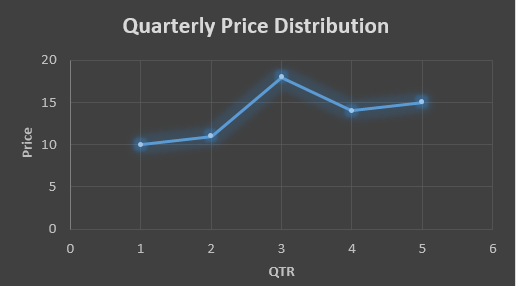….fig (1)

The plot above shows that there is no trend the data is following so to better understand the pattern we calculate the moving average over three quarter at a time so that we get in between values as well as we get the missing value of the 6th quarter.

To find the missing value of 6th quarter we will use previous three quarter’s data i.e.

MAS =  = 15.7

 QTR (quarter) Price 1 10 2 11 3 18 4 14 5 15 6 15.7

MAS =  = 13

MAS =  = 14.33

 QTR (quarter) Price MAS (Price) 1 10 10 2 11 11 3 18 18 4 14 13 5 15 14.33 6 15.7 15.7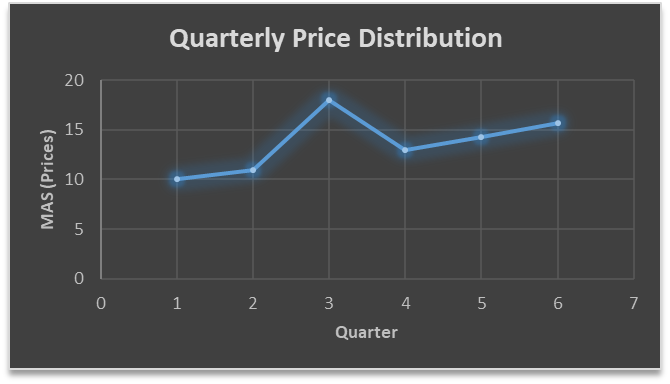….. fig (2)

In the above graph we can see that after 3rd quarter there is an upward sloping trend in the data.

Exponential Data Smoothing

In this method a larger weight ( ) which lies between 0 & 1 is given to the most recent observations and as the observation grows more distant the weight decreases exponentially.

The weights are decided on the basis how the data is, in case the data has low movement then we will choose the value of  closer to 0 and in case the data has a lot more randomness then in that case we would like to choose the value of  closer to 1.

EMA= Ft= Ft-1 + (At-1 – Ft-1)

Now lets see a practical example.

For this example we will be taking  = 0.5

Taking the same data……

 QTR (quarter) Price(At) EMS Price(Ft) 1 10 10 2 11 ? 3 18 ? 4 14 ? 5 15 ? 6 ? ?

To find the value of yellow cell we need to find out the value of all the blue cells and since we do not have the initial value of F1 we will use the value of A1. Now lets do the calculation:-

F2=10+0.5(10 – 10) = 10

F3=10+0.5(11 – 10) = 10.5

F4=10.5+0.5(18 – 10.5) = 14.25

F5=14.25+0.5(14 – 14.25) = 14.13

F6=14.13+0.5(15 – 14.13)= 14.56

 QTR (quarter) Price(At) EMS Price(Ft) 1 10 10 2 11 10 3 18 10.5 4 14 14.25 5 15 14.13 6 14.56 14.56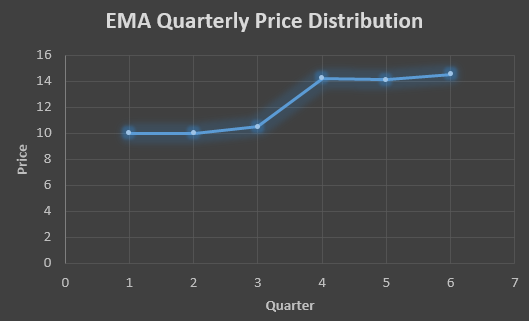In the above graph we see that there is a trend now where the data is moving in the upward direction.

So, with that we come to the end of the discussion on the Data smoothing method. Hopefully it helped you understand the topic, for more information you can also watch the video tutorial attached down this blog. The blog is designed and prepared by Niharika Rai, Analytics Consultant, DexLab Analytics DexLab Analytics offers machine learning courses in Gurgaon. To keep on learning more, follow DexLab Analytics blog.

.

## Time Series Analysis Part I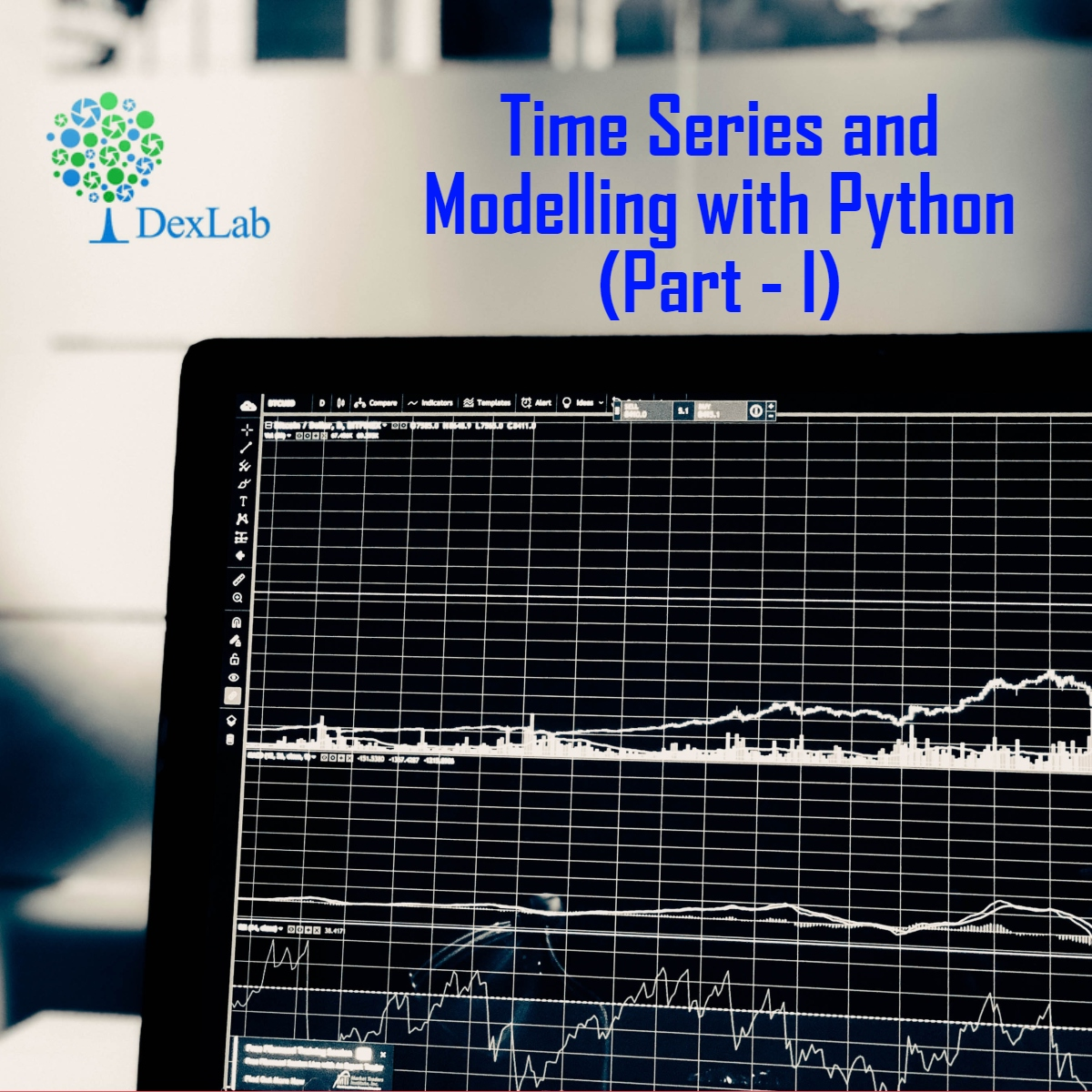A time series is a sequence of numerical data in which each item is associated with a particular instant in time. Many sets of data appear as time series: a monthly sequence of the quantity of goods shipped from a factory, a weekly series of the number of road accidents, daily rainfall amounts, hourly observations made on the yield of a chemical process, and so on. Examples of time series abound in such fields as economics, business, engineering, the natural sciences (especially geophysics and meteorology), and the social sciences.

• Univariate time series analysis- When we have a single sequence of data observed over time then it is called univariate time series analysis.
• Multivariate time series analysis – When we have several sets of data for the same sequence of time periods to observe then it is called multivariate time series analysis.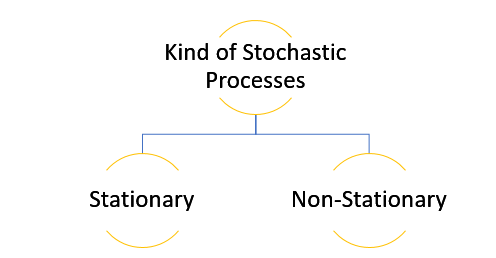The data used in time series analysis is a random variable (Yt) where t is denoted as time and such a collection of random variables ordered in time is called random or stochastic process.

Stationary: A time series is said to be stationary when all the moments of its probability distribution i.e. mean, variance , covariance etc. are invariant over time. It becomes quite easy forecast data in this kind of situation as the hidden patterns are recognizable which make predictions easy.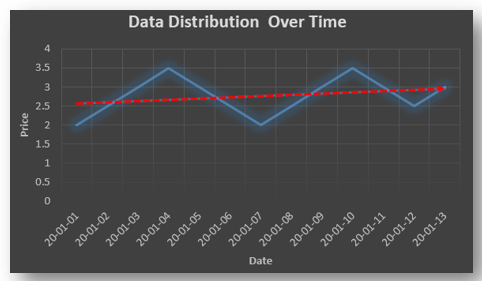Non-stationary: A non-stationary time series will have a time varying mean or time varying variance or both, which makes it impossible to generalize the time series over other time periods.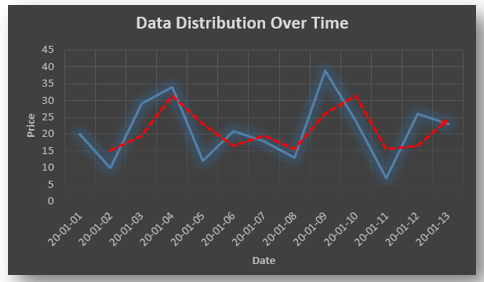Non stationary processes can further be explained with the help of a term called Random walk models. This term or theory usually is used in stock market which assumes that stock prices are independent of each other over time. Now there are two types of random walks:
Random walk with drift : When the observation that is to be predicted at a time ‘t’ is equal to last period’s value plus a constant or a drift (α) and the residual term (ε). It can be written as
Yt= α + Yt-1 + εt
The equation shows that Yt drifts upwards or downwards depending upon α being positive or negative and the mean and the variance also increases over time.
Random walk without drift: The random walk without a drift model observes that the values to be predicted at time ‘t’ is equal to last past period’s value plus a random shock.
Yt= Yt-1 + εt
Consider that the effect in one unit shock then the process started at some time 0 with a value of Y0
When t=1
Y1= Y0 + ε1
When t=2
Y2= Y1+ ε2= Y0 + ε1+ ε2
In general,
Yt= Y0+∑ εt
In this case as t increases the variance increases indefinitely whereas the mean value of Y is equal to its initial or starting value. Therefore the random walk model without drift is a non-stationary process.

So, with that we come to the end of the discussion on the Time Series. Hopefully it helped you understand time Series, for more information you can also watch the video tutorial attached down this blog. DexLab Analytics offers machine learning courses in delhi. To keep on learning more, follow DexLab Analytics blog.

.

## An Introductory Guide to NumPy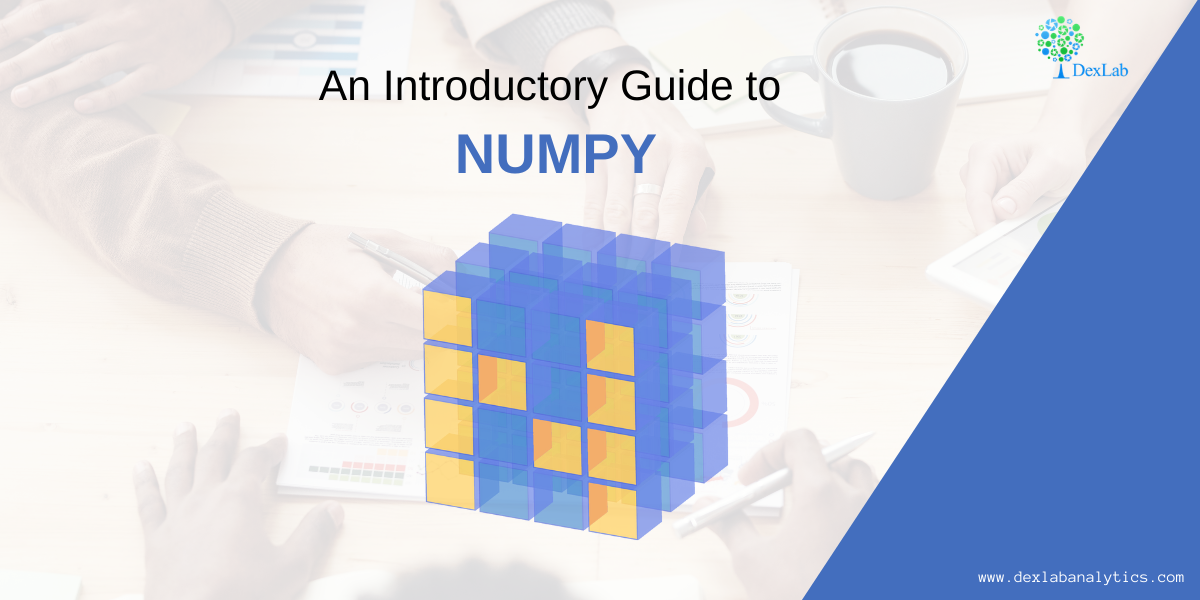NumPy also known as numerical python, is a library consisting of multidimensional array objects and a collection of routines for processing those arrays. Using NumPy, mathematical and logical operations on arrays can be performed without it which was not possible. For example-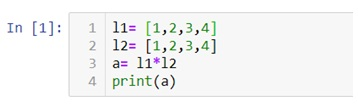Multiplication of two lists will cause an error as a data structure like lists, tuple, dictionaries and sets do not allow mathematical operations.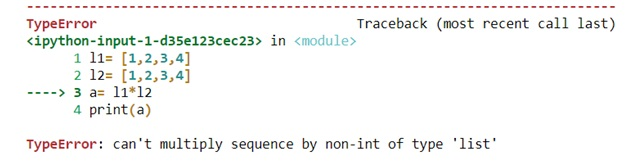Therefore we need NumPy to covert our data structures like lists into 1d, 2d, 3d or nd arrays so that mathematical operations can be performed. U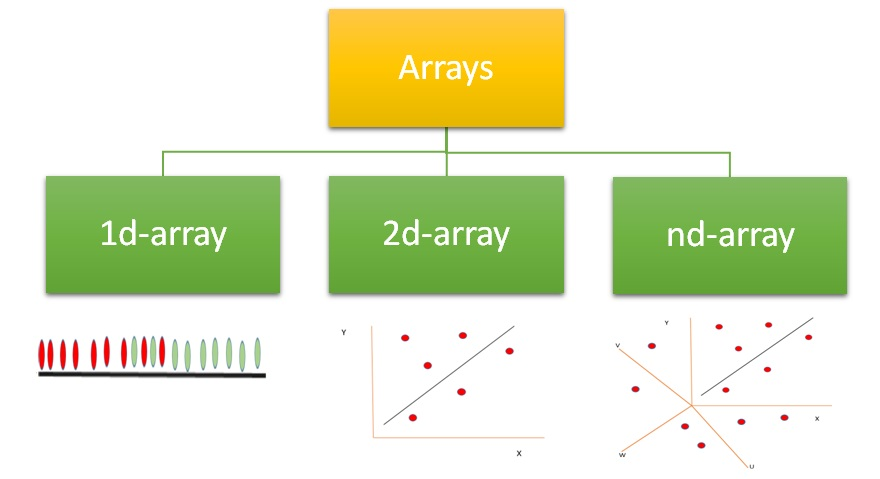We can use .array() methods to create these arrays.

Now let’s check out few examples and also perform few mathematical operations to have a better understanding.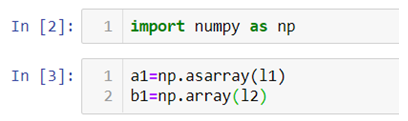Arrays can be created using lists, tuples and dictionaries as you can see in the above example.

Now for 2-d arrays recall that we can also make list of lists. Let’s use that to create 2d-arrays.

2d-arrays can also be created using tuples.

Remember that we are not using these as matrices because matrix multiplication is an entirely different thing we are just trying to perform mathematical operations which were otherwise not possible.

#### Random Module

Numpy also has various ways with which we can create array of random numbers which then can be used in number of ways like generating a data for practice purposes or for building beautiful graphs for a presentation.

Given below is a list of type of random numbers you can generate

.rand() :- This particular method helps you generate uniformly distributed random numbers i.e. numbers between 0 and 1 where each number between 0 and 1 will have equal probability to be in the sample dataset.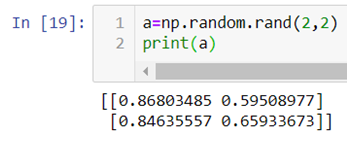The above code generates a 2d-array with values between 0 and 1.

.randn():- This method generates normally distributed random numbers i.e. numbers between -3 and +3 where mean=median=mode and ploted gives a bell shaped curve.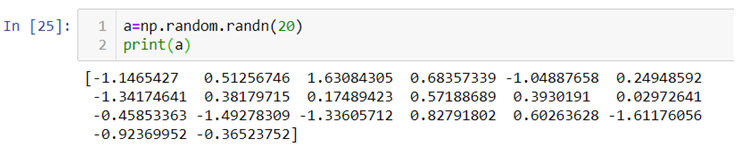Here the 20 random numbers are generated ranging between -3 and + 3.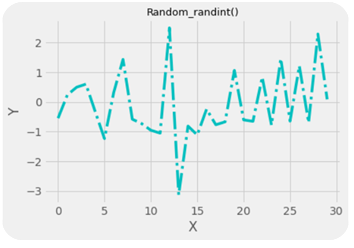Note:- Remember that the data is randomly picked from the normally distributed values between -3 and +3 so the graph is not bell shaped but the original data from which the values are being picked randomly is bell shaped with mean=median-mode.

.randint():-This method generates random integers between a given range.

So, with that we come to the end of the discussion on the Numpy. Hopefully it helped you understand Numpy, for more information you can also watch the video tutorial attached down this blog. DexLab Analytics offers machine learning courses in delhi. To keep on learning more, follow DexLab Analytics blog.

.

## 5 Ways Artificial Intelligence Will Impact Our FutureArtificial Intelligence, or, its more popular acronym AI is no longer a term to be read about in a sci-fi book, it is a reality that is reshaping the world by introducing us to virtual assistants, helping us be more secure by enabling us with futuristic measures. The evolution of AI has been pretty consistent and as we are busy navigating through a pandemic-ridden path towards the future, adapting to the “new normal”, and becoming increasingly reliant on technology, AI assumes a greater significance.

The AI applications which are already being implemented has resulted in a big shift, causing an apprehension that the adoption of AI technology on a larger scale would eventually lead to job cuts, whereas in reality, it would lead to the creation of new jobs across industries. Adoption of AI technology would push the demand for a workforce that is highly skilled, enrolling in an artificial intelligence course in delhi could be a timely decision.

Now that we are about to reach the end of 2020, let us take a look at the possible impacts of AI in the future.

#### AI will create more jobs

Yes, contrary to the popular apprehension AI would end up creating jobs in the future. However, the adoption of AI to automate tasks means yes, there would be a shift, and a job that does not need special skills will be handled by AI powered tools. Jobs that could be done without error, completed faster, with a higher level of efficiency, in short better than humans could be performed by robots. However, with that being said there would be more specialized job roles, remember AI technology is about the simulation of human intelligence, it is not the intelligence, so there would be humans in charge of carrying out the AI operated areas to monitor the work. Not just that but for developing smarter AI application and implementation there should be a skilled workforce ready, a report by World Economic Forum is indicative of that. From design to maintenance, AI specialists would be in high demand especially the developers. The fourth industrial revolution is here, industries are gearing up to build AI infrastructure, it is time to smell the coffee as by the end of 2022 there will be millions of AI jobs waiting for the right candidates.

#### Dangerous jobs will be handled by robots

In the future, hazardous works will be handled by robots. Now the robots are already being employed to handle heavy lifting tasks, along with handling the mundane ones that require only repetition and manual labor. Along with automating these tasks, the robot workforce can also handle the situation where human workers might sustain grave injuries. If you have been aware and interested then you already heard about the “SmokeBot”. In the future, it might be the robots who will enter the flaming buildings for assessment before their human counterparts can start their task. Manufacturing plants that deal with toxic elements need robot workers, as humans run a bigger risk when they are exposed to such chemicals. Furthermore, the nuclear plants might have a robot crew that could efficiently handle such tasks. Other areas like pipeline exploration, bomb defusing, conducting rescue operations in hostile terrain should be handled by AI robots.

#### Smarter healthcare facilities

AI  implementation which has already begun would continue to transform the healthcare services. With AI being in place CT scan and MRI images could be more precise pointing out even minuscule changes that earlier went undetected. Drug development could also be another area that would see vast improvement and in a post-pandemic world, people would need to be better prepared to fight against such viruses. Real-time detection could prevent many health issues going severe and keeping a track of the health records preventive measures could be taken. One of the most crucial changes that could be revolutionary, is the personalized medication which could only be driven by AI technology. This would completely change the way healthcare functions. Now that we are seeing chat bots for handling sales queries, the future healthcare landscape might be ruled by virtual assistants specifically developed for offering assistance to the patients. There are going to be revolutionary changes in this field in the future, thereby pushing the demand for professionals skilled in deep learning for computer vision with python.

#### Smarter finance

We are already living in an age where we have robo advisors, this is just the beginning and the growing AI implementation would enable an even smarter analytics system that would minimize the credit risk and would allow banks and other financial institutes to minimize the risk of fraud. Smarter asset management, enhanced customer support are going to be the core features. Smarter ML algorithms would detect any and every oddity in behavior or in transactions and would help prevent any kind of fraud from happening. With analytics being in place it would be easier to predict the future trends and thereby being more efficient in servicing the customers. The introduction of personalized services is going to be another key feature to look out for.#### Retail space gets a boost

The retailers are now aiming to implement AI applications to  offer smart shopping solutions to the future buyers. Along with coming up with personalized shopping suggestions for the customers and showing them suggestions based on their shopping pattern, the retailers would also be using the AI to predict the future trends and work accordingly. Not just that but they can easily maintain the supply and demand balance with the help of AI solutions and stock up items that are going to be in demand instead of items that would not be trendy. The smarter assistants would ensure that the customer queries are being handled and they could also be helping them with shopping by providing suggestions and information. From smart marketing to smarter delivery, the future of retail would be dominated by AI as the investment in this space is gradually going up.

The future is definitely going to be impacted by the AI technology in more ways than one. So, be future ready and get yourself upskilled as it is the need of the hour, stay updated and develop the skill to move towards the AI future with confidence.

.

## Linear Regression Part I: A Comprehensive Guide to Linear Regression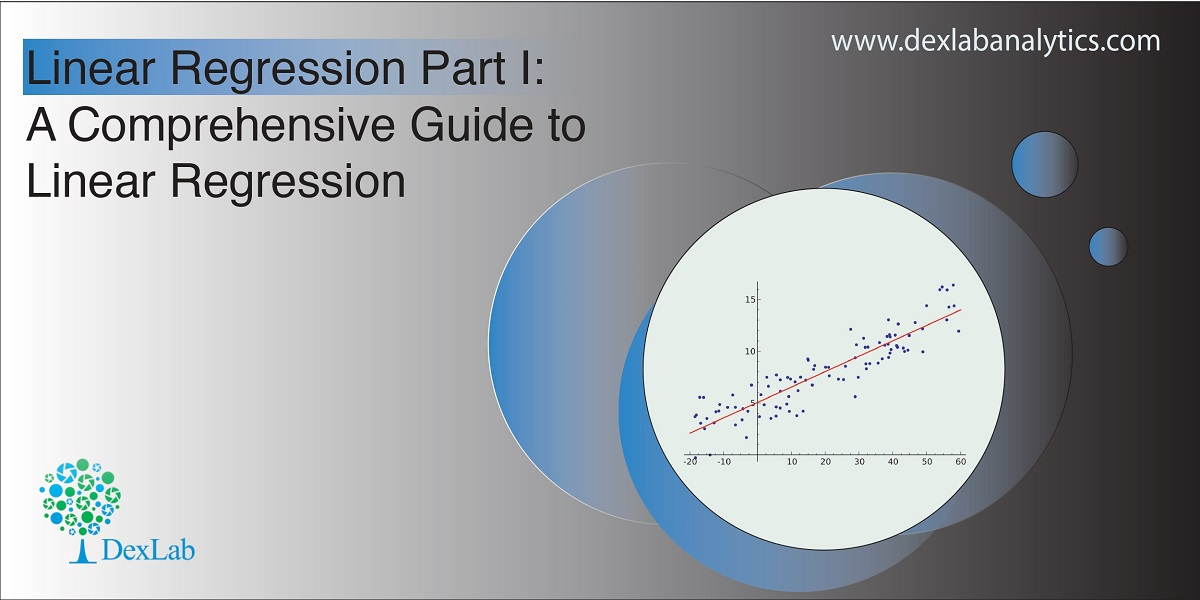Today’s blog explores another vital statistical concept Linear Regression, let’s begin. Linear regression is normally used in statistics for predictive modeling. It tries to model a relationship between two independent (explanatory variable) and dependent (explained variable) variables X and Y by fitting a linear equation (Y=bo+b1X+Ui) to an observed data.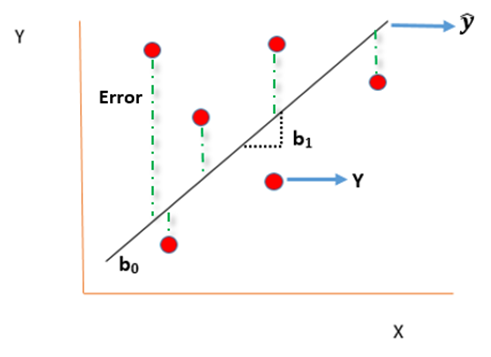#### Assumptions of linear regression

• Ui is a random real variable, where Ui is the difference between the observed dependent variable Y and predicted Y variable.
• The mean of Ui in any particular period is zero.
• The variance of Ui is constant in each period i.e for all values of X, Ui will show the same dispersion around their mean
• The variable Ui has a normal distribution i.e the value of Ui (for each Xi) have a bell shaped symmetrical distribution about their zero mean.
• The random terms of different observations are independent i.e the covariance of any Ui with any other Uj is equal to zero.
• Ui is independent of the explanatory variable X.
• Xi are a set of fixed values in the hypothesised process of repeated sampling which underlies the linear regression model.
• In case there are more than one explanatory variables then they are not perfectly linearly correlated.

Linear Regression equation can be written as: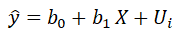Where,

is the dependent variable

X is the independent variable.

b0 is the intercept (where the line crosses the vertical y-axis)

b1 is the slope

Ui is the error term (difference between ) also called residual or white noise.#### Simple linear regression follows the properties of Ordinary Least Square (OLS) which are as follows:-

1. Unbiased estimator:- E()=b ie. an estimator is unbiased if its bias is 0; E() – b = 0
2. Minimum Variance:- An estimate is best when it has the smallest variance as compared to any other estimate obtained from other econometric method.
3. Efficient estimator:- When it has both the previous properties ie.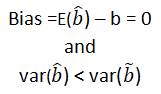4. Linear estimator
5. Best, Linear, Unbiased estimator (BLUE)
6. Minimum mean squared error (MSE) estimator:- It is a combination of the unbiasedness and minimum variance properties. An estimator is a minimum MSE estimator if it has the smallest mean square error.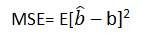With that the discussion on Linear Regression wraps up here, hopefully it cleared away any confusion you might have and helped you get a grasp on the concept. We have a video discussion on this same topic, which is attached below this blog, check it out for further reference.

Continue to track the DexLab Analytics blog to find informative posts related to Python for data science training.

.

+91 931 572 5902